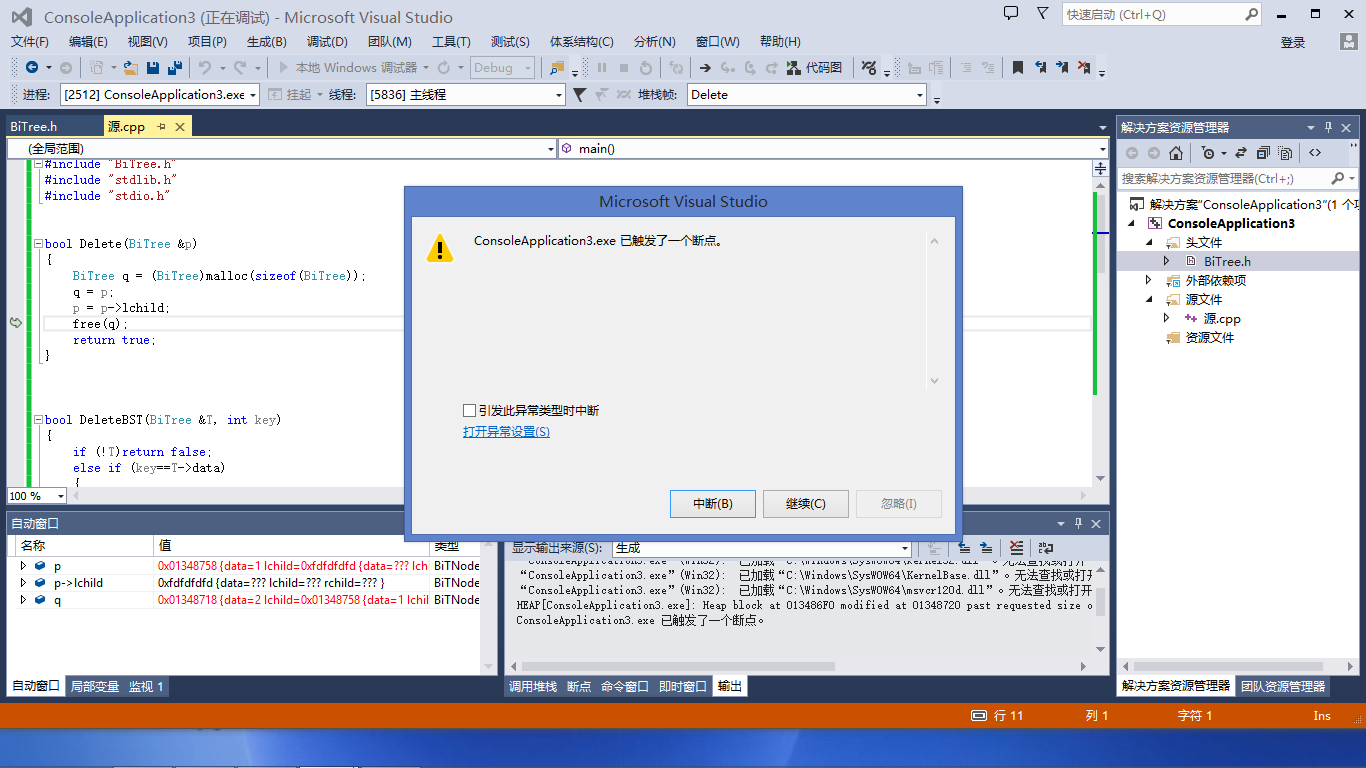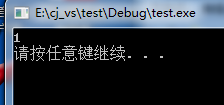c语言，free总是提示触发了断点，求指导。

`````` typedef struct BiTNode
{
int data;
struct BiTNode *lchild, *rchild;

}BiTNode, *BiTree;
``````

`````` #include "BiTree.h"
#include "stdlib.h"
#include "stdio.h"

bool Delete(BiTree &p)
{
BiTree q = (BiTree)malloc(sizeof(BiTree));
q = p;
p = p->lchild;
free(q);
return true;
}

bool DeleteBST(BiTree &T, int key)
{
if (!T)return false;
else if (key==T->data)
{
return Delete(T);
}
else if (key < T->data)
{
return DeleteBST(T->lchild, key);
}
}

int main()
{
BiTree t1 = (BiTree)malloc(sizeof(BiTree));
BiTree t2 = (BiTree)malloc(sizeof(BiTree));
BiTree t3 = (BiTree)malloc(sizeof(BiTree));
t1->data = 3;
t2->data = 2;
t3->data = 1;
t1->lchild = t2;
t1->rchild = NULL;
t2->lchild = t3;
t2->rchild = NULL;
DeleteBST(t1, 2);

printf("%d\n", t1->lchild->data);

system("PAUSE");

return 0;

}

``````3个回答

``````  #include "BiTree.h"
#include "stdlib.h"
#include "stdio.h"

bool Delete(BiTree &p)
{
BiTree q = (BiTree)malloc(sizeof(BiTNode));
q = p;
p = p->lchild;
free(q);
return true;
}

bool DeleteBST(BiTree &T, int key)
{
if (!T)return false;
else if (key==T->data)
{
return Delete(T);
}
else if (key < T->data)
{
return DeleteBST(T->lchild, key);
}
}

int main()
{
BiTree t1 = (BiTree)malloc(sizeof(BiTNode));
BiTree t2 = (BiTree)malloc(sizeof(BiTNode));
BiTree t3 = (BiTree)malloc(sizeof(BiTNode));
t1->data = 3;
t2->data = 2;
t3->data = 1;
t1->lchild = t2;
t1->rchild = NULL;
t2->lchild = t3;
t2->rchild = NULL;
DeleteBST(t1, 2);

printf("%d\n", t1->lchild->data);

system("PAUSE");

return 0;

}

``````c语言链栈运行时触发断点求解求解

#include<stdio.h> #include<stdlib.h> #define P1 #ifdef P1 typedef struct { char name; int age; }DataType; typedef struct stacknode { DataType data; struct stacknode *next; }StackNode; typedef struct { StackNode *top; }LinkStack; StackNode *StackInit(void) { StackNode *p; if (p = ((StackNode *)malloc(sizeof(StackNode)))) { p->next = NULL; return p; } return NULL; } void InitStack(LinkStack *s) { s->top = NULL; } int StackEmpty(LinkStack *s) { return s->top == NULL; } void Push(LinkStack *s, DataType x) { StackNode *p = (StackNode *)malloc(sizeof(StackNode)); InitStack(s); p -> data = x; p->next = s->top; s->top = p; } DataType Pop(LinkStack *s) { DataType x; StackNode *p = s; if (StackEmpty(s)) { printf("stack underflow"); } x = p->data; s->top = p->next; free(p); return x; } DataType StackTop(LinkStack *s) { if (StackEmpty(s)) { printf("stack is empty"); } return s->top->data; } int main(void) { StackNode *stack; DataType data, data1; stack = StackInit(); printf("接下来进行入栈操作!\n"); printf("按照 姓名 年龄依次输入信息: "); scanf("%s%d", data.name, &data.age); Push(stack, data); printf("按照 姓名 年龄依次输入信息: "); scanf("%s%d", data.name, &data.age); Push(stack, data); printf("接下来进行出栈操作!\n"); data1 = Pop(stack); printf("出栈操作的数据是(%s,%d)\n", data1.name, data1.age); data1 = Pop(stack); printf("出栈操作的数据是(%s,%d)\n", data1.name, data1.age); system("pause"); return 0; } #endif

C语言free问题，出现运行时错误CRT

C语言中Free函数释放内存为何释放不了！！！！！！！

#include <stdafx.h> #include<string> void testFree(char *par) { void*free(par); par = NULL; } int _tmain(int argc, _TCHAR* argv[]) { char path = {"abc"}; char *str = (char *) malloc(100 *sizeof(char)); memset(str,0x00,100 *sizeof(char)); memcpy(str,path,strlen(path)); printf("wwl1:%s\n",str); testFree(str); printf("wwl2:%s\n",str); return 0; } 打印结果为： wwl1:abc wwl2:abc 正常的话不是应该为：wwl1:abc wwl2:(null) d 的么？为何输出有误，为何str内存并未释放？testFree函数地址传递有误么？

C语言断点调试VC6.0有没有大神帮忙看下

c语言 求个导数 怎么也做不对 求帮助

Description 给定函数：y = ( 1 + x2 )x。 针对输入变量x的取值，计算y'（y的导函数）的值。 Input 输入的第一个为整数N，后接N个变量x的取值。 Output 对每个输入的x值，每行输出一个y'的值，保留2位有效数字。 Sample Input 2 1 0.6 Sample Output 3.39 1.01 HINT 先求导数吧，然后把x代入y'。

C语言统计之众数，新手求大神指教，多谢！

C语言中malloc和free的问题

int *intPointer = (int *)malloc(3 * sizeof(int)); intPointer = 1; intPointer = 2; intPointer = 3; printf("before free :%ld\n",intPointer); free(intPointer); printf("after free :%ld\n",intPointer); printf("%d\t%d\t%d\n",intPointer,intPointer,intPointer); 输出结果： before free :4296037472 after free :4296037472 1 2 3 疑问：free(intPointer)之后，为什么还能通过intPointer访问malloc分配的那片内存？

C语言中如何调试函数求指导

C语言中如何调试函数?按F11几步之后进入了如下图一些文件中开始逐步调试，这是怎么回事？ 就是一些诸如printf.c, _file.c的文件，怎么能跳过这些，调试函数呢？

C语言中指针释放问题，函数free()的使用。

c语言学习基础差，求指导

C语言怎么用递归求组合数

C语言用递归求圆周率的值，怎么实现

C语言用递归求圆周率的值，要求精确到小数点后3位，不得使用循环

c语言简单输出问题，求指导

#include <stdio.h> #include <stdlib.h> main () { int a=6,b=7; printf("%d,%d\n",a,b); { int b=10; printf("%d,%d\n",a,b); } printf("%d,%d\n",a,b); } 输出结果是：6，7 6，10 6，7 我不明白为什么打三个输出是6，7 求指导，自学c语言，没有老师问

String s = new String(" a ") 到底产生几个对象？

Linux面试题（2020最新版）

JVM内存结构和Java内存模型别再傻傻分不清了

JVM内存结构和Java内存模型都是面试的热点问题，名字看感觉都差不多，网上有些博客也都把这两个概念混着用，实际上他们之间差别还是挺大的。 通俗点说，JVM内存结构是与JVM的内部存储结构相关，而Java内存模型是与多线程编程相关，本文针对这两个总是被混用的概念展开讲解。 JVM内存结构 JVM构成 说到JVM内存结构，就不会只是说内存结构的5个分区，而是会延展到整个JVM相关的问题，所以先了解下

loonggg读完需要3分钟速读仅需 1 分钟大家好，我是你们的校长。我之前讲过，这年头，只要肯动脑，肯行动，程序员凭借自己的技术，赚钱的方式还是有很多种的。仅仅靠在公司出卖自己的劳动时...

85后蒋凡：28岁实现财务自由、34岁成为阿里万亿电商帝国双掌门，他的人生底层逻辑是什么？...

MySQL数据库面试题（2020最新版）

HashMap底层实现原理，红黑树，B+树，B树的结构原理 Spring的AOP和IOC是什么？它们常见的使用场景有哪些？Spring事务，事务的属性，传播行为，数据库隔离级别 Spring和SpringMVC，MyBatis以及SpringBoot的注解分别有哪些？SpringMVC的工作原理，SpringBoot框架的优点，MyBatis框架的优点 SpringCould组件有哪些，他们...